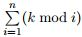Competitions

# Josephs Problem

Time limit 1 second
Memory limit 64 MiB

Joseph likes taking part in programming contests. His favorite problem is, of course, Joseph’s problem. It is stated as follows.

There are n persons numbered from 0 to n−1 standing in a circle. The person number k, counting from the person number 0, is executed. After that the person number k of the remaining persons is executed, counting from the person after the last executed one. The process continues until only one person is left. This person is a survivor. The problem is, given n and k detect the survivor’s number in the original circle.

Of course, all of you know the way to solve this problem. The solution is very short, all you need is one cycle:

r := 0;

for i from 1 to n do

r := (r + k) mod i;

return r;

Here "x mod y" is the remainder of the division of x by y.

But Joseph is not very smart. He learned the algorithm, but did not learn the reasoning behind it. Thus he has forgotten the details of the algorithm and remembers the solution just approximately.

He told his friend Andrew about the problem, but claimed that the solution can be found using the following algorithm:

r := 0;

for i from 1 to n do

r := r + (k mod i);

return r;

Of course, Andrew pointed out that Joseph was wrong. But calculating the function Joseph described is also very interesting.Given n and k, ﬁnd .

## Input data

The input ﬁle contains n and k (1 ≤ nk ≤ 109).

## Output data

Output the sum requested.

## Examples

Input example #1
5 3

Output example #1
7
`